# Let's try something more advanced now!

Creating a whole game is not an easy task. There are a lot of little details that need to be considered to make the game work as good as possible. When creating your own game, always set the goals at the beginning so that you can work from the bottom up with some structure in mind. Writing code without a bigger picture in mind can be a big problem later in code development.

The game that we'll create will showcase pretty much every feature we've gone through so far.

It will look like a simple video game, where you'll move a character that collects objects while counting a score.

Let's begin!
Now, let's make some variables and name them "posX" and "posY". Those will define your (player's) position on the screen.Drag and drop them onto the drawing area.Find this block named "123" in the "Math" section. Type in the numerical value you want once you drop it onto the drawing area.

Write these numerical values in the circles:Create another set of variables that'll determine the speed of your ball.

Let's call them "velocityX" and "velocityY". Drag and drop the new variables under the variables we used for determining position.

"VelocityX" means that this will be the velocity of the ball in the x-axis direction, and "velocityY" determines the velocity of the ball in the y-axis direction.

Make the numerical value of the velocity 0.01 for both X and Y.Let's make a few more variables while setting up our game.

You can name them "lastTime" and "score". Of course, you should put the value of the "score" to zero (0) at the beginning of the code.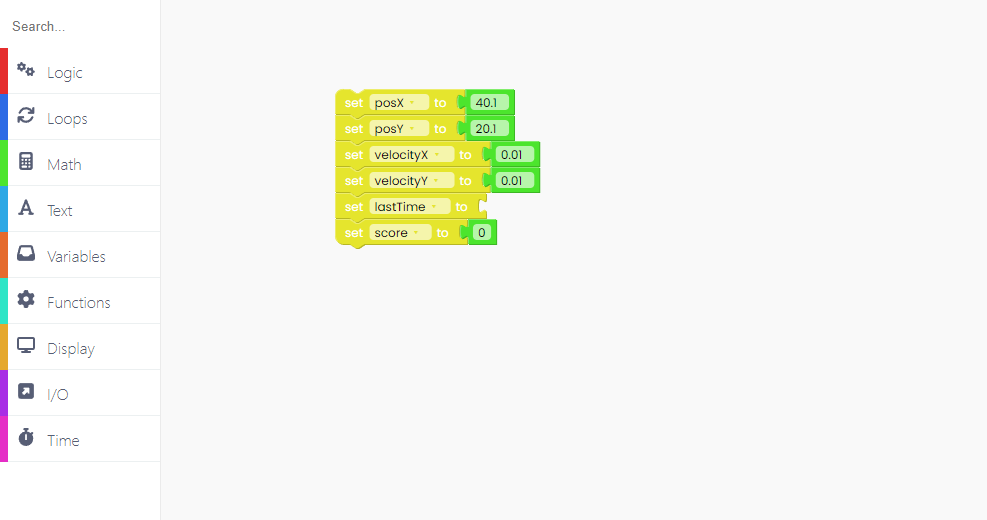Now go to the Time block section and look for the "ms since started" block and add it to the "lastTime" variable.We have to create a function if we want to execute some code.

Let's name our function "createPickup" and place it under the "set score" variable.You probably guessed the next step - creating variables for the newly made function.

Let's call them "pickupX" and "pickupY" as they'll define the position of the pickup (the smaller ball you'll have to catch).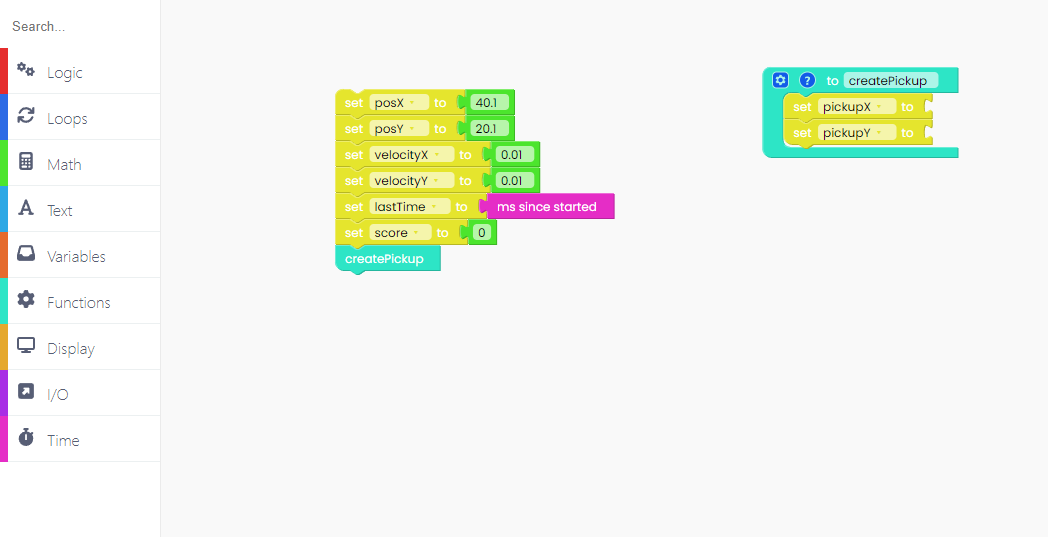We'll set those variables to a random integer, and you'll find that block in the Math section.

An integer is a whole number that can be positive, negative, or zero. Examples of the integer are: '5, 1, 5, 8, 97.

Place those blocks in the pickup variables, and write the numerical value.

For "pickups" and "pickupY" you have to use a random number from 0 to 150 because the display resolution is 160*120 pixels.Firstly, we'll create a new function and call it "physics".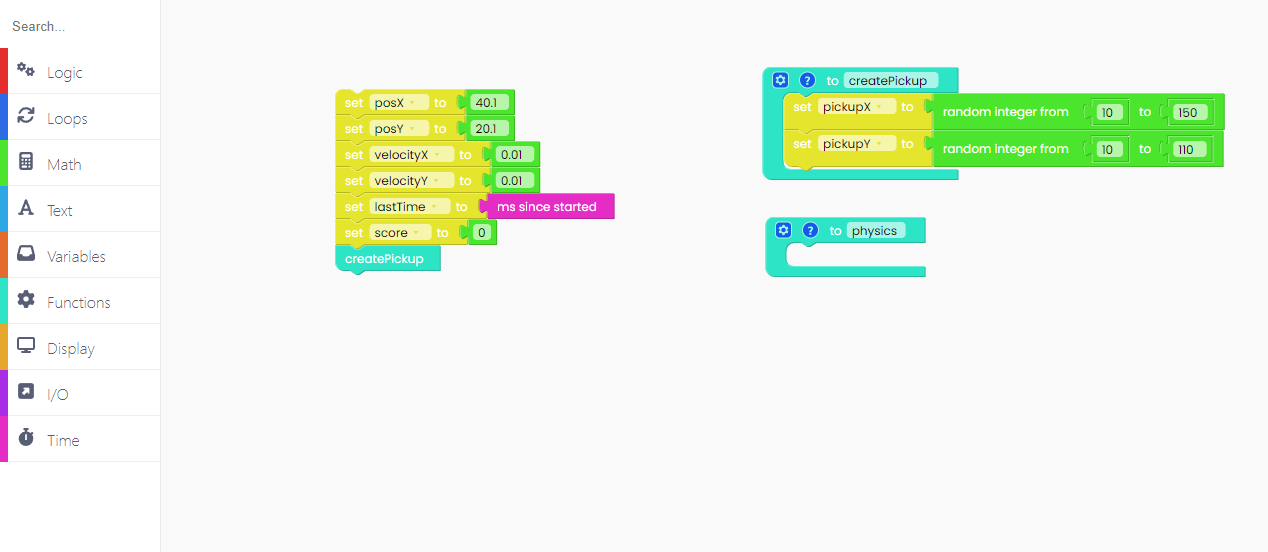The first thing we have to do in the physics function is create a new variable called "timeDelta".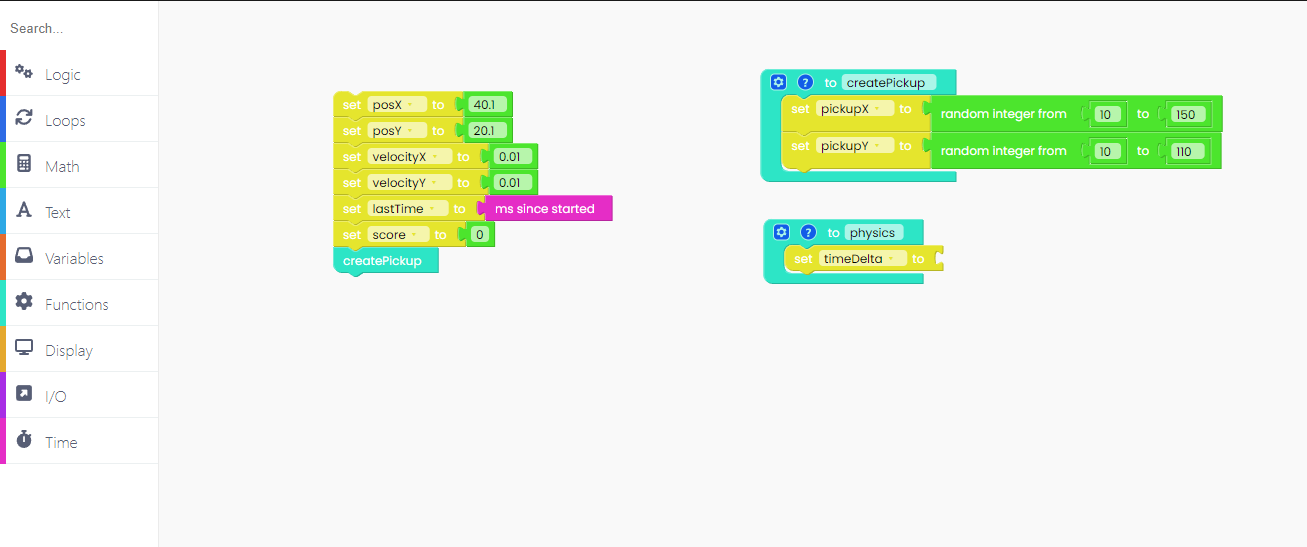A Greek symbol delta is used to note the difference between the two things. We created the variable "timeDelta" to note the elapsed and measured time
difference.

We will calculate "timeDelta" by subtracting from the current time the last time the function was executed. Also, we'll have to divide it by 1000.01 to get the time in seconds.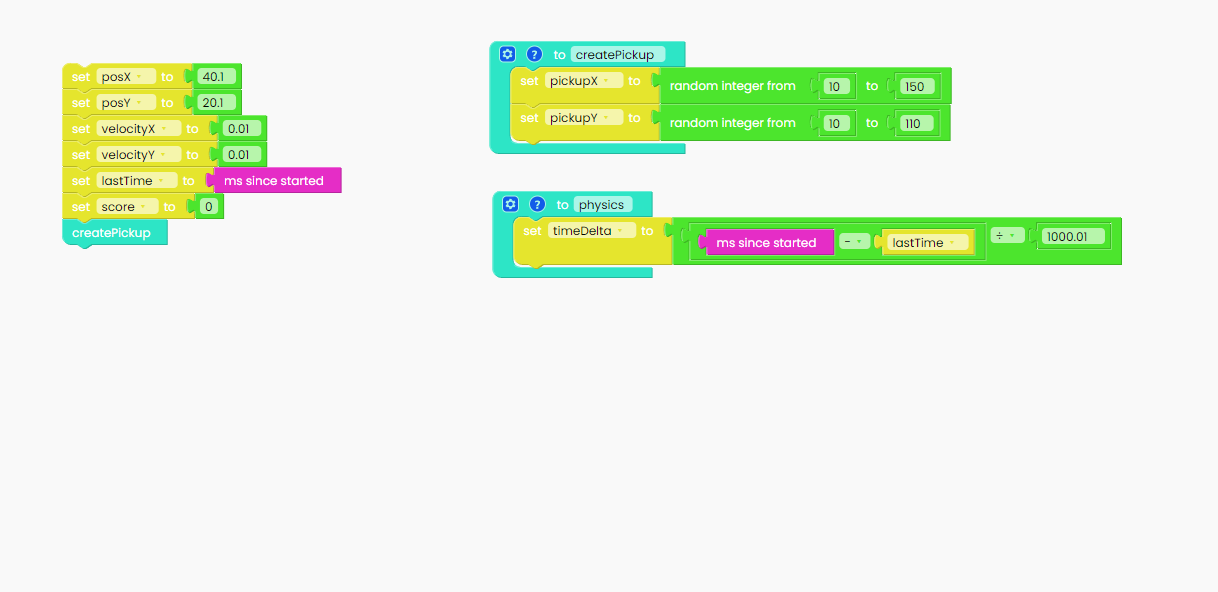Now, let's play with the physics formulas a little bit.

"VelocityY" will increase by multiplication of "timeDelta" and 60.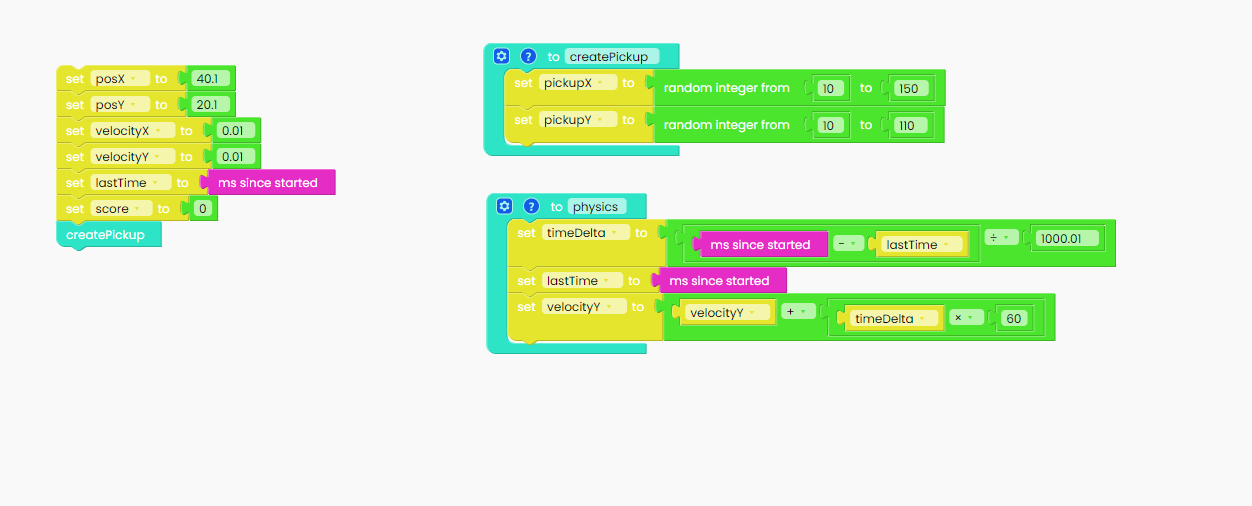We have to update position X and position Y based on velocity.

You can do that by using "change posX/posY" blocks and placing them under the "change velocityY" block.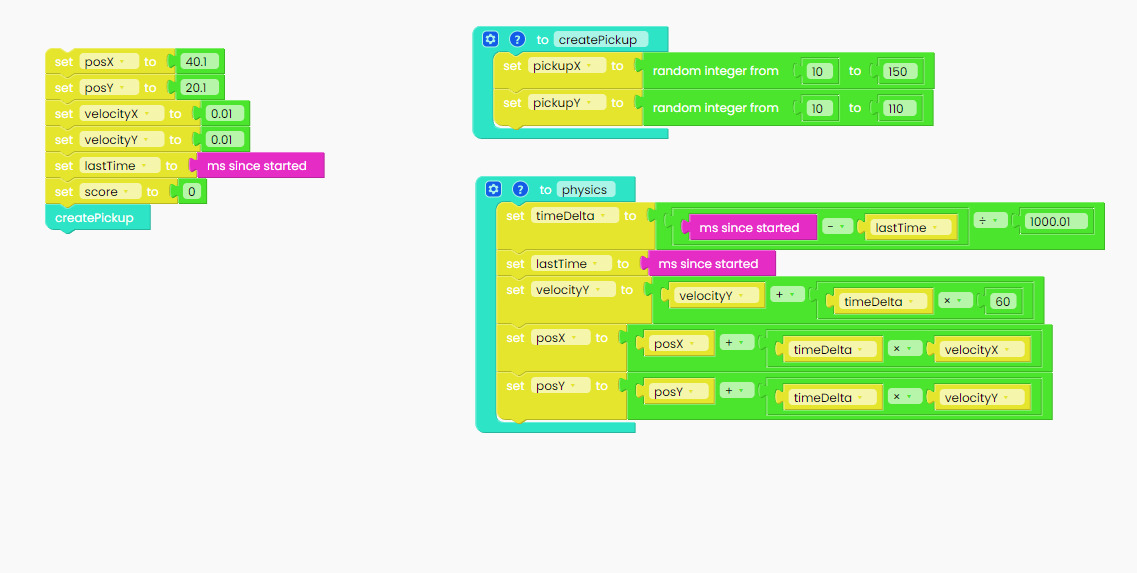Now, let's see what will happen if the ball hits one of the edges of the display.

We'll use the "if-do-else" block from the Logic section and place the comparison block in it.

Your code will check if the ball's position on the x-axis is under five (5). As we mentioned before, the x-axis goes from 0 to 150.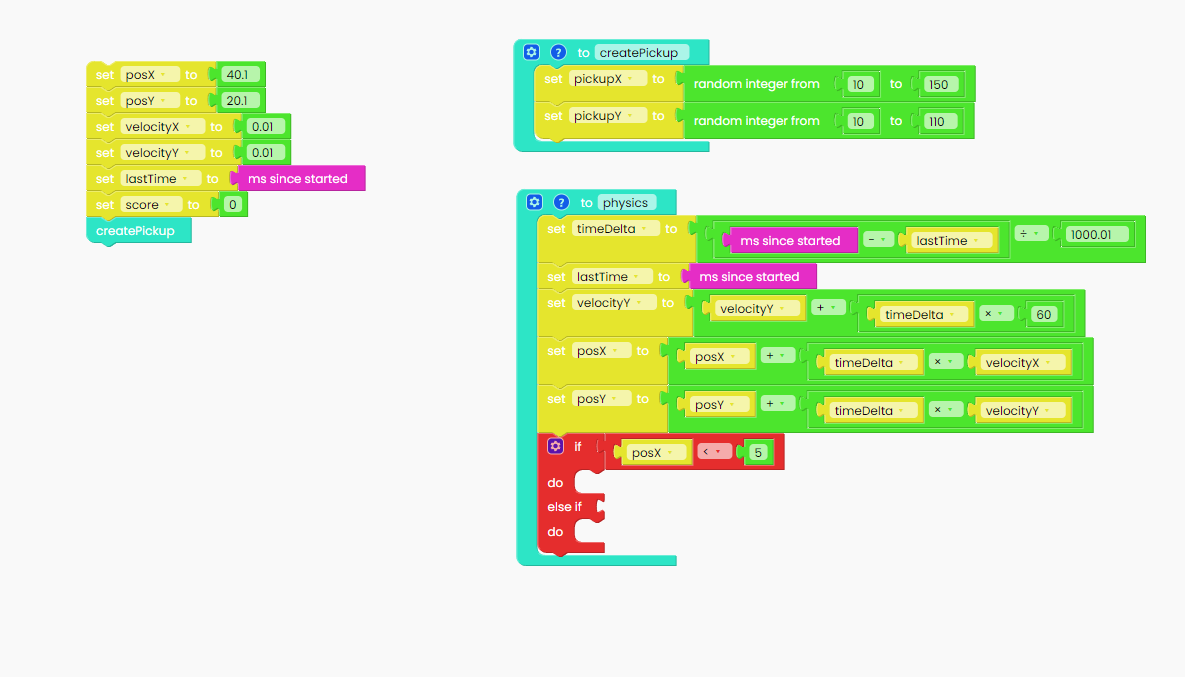If position X is under the numerical value we gave it, which is five, velocityX will change into -velocityX (negative on the axis)

In other words, the ball will change its direction when it hits the edge.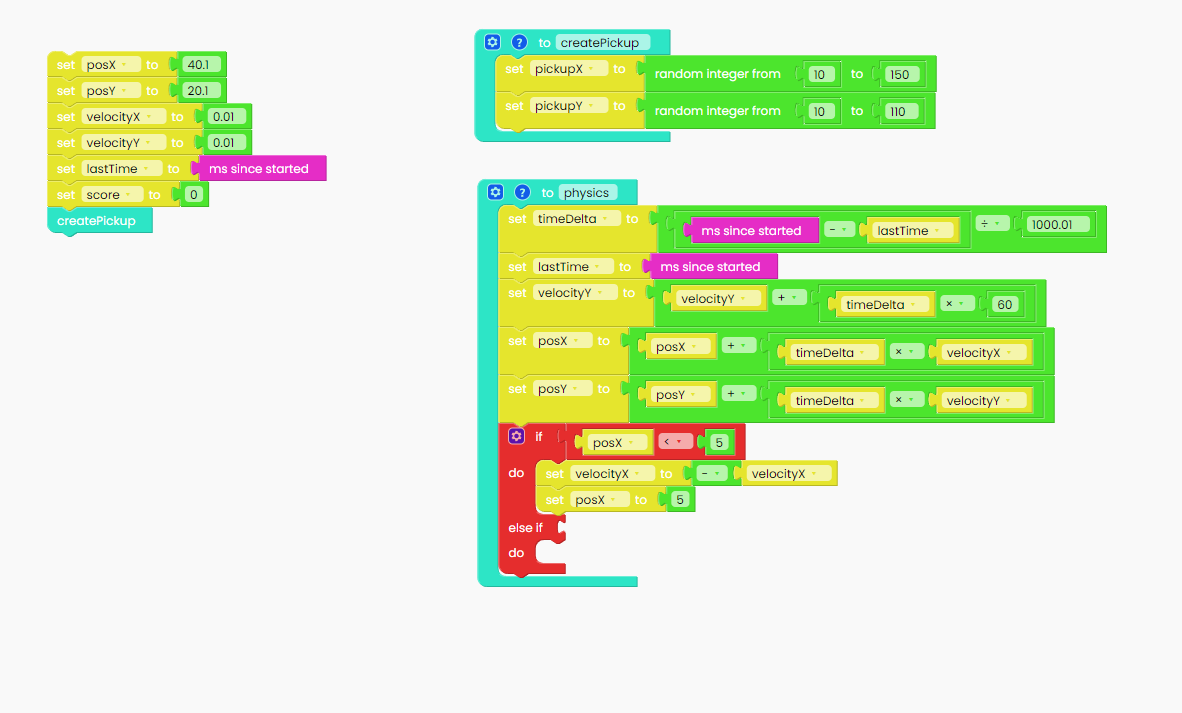Now, let's see what happens if the ball is in position over 155.

Once again, we have decided that the posX is set to 155. So, if the velocityX is bigger than 155, the ball will change its direction.Now, let's repeat the procedure for the Y position.

The physics function should look like this: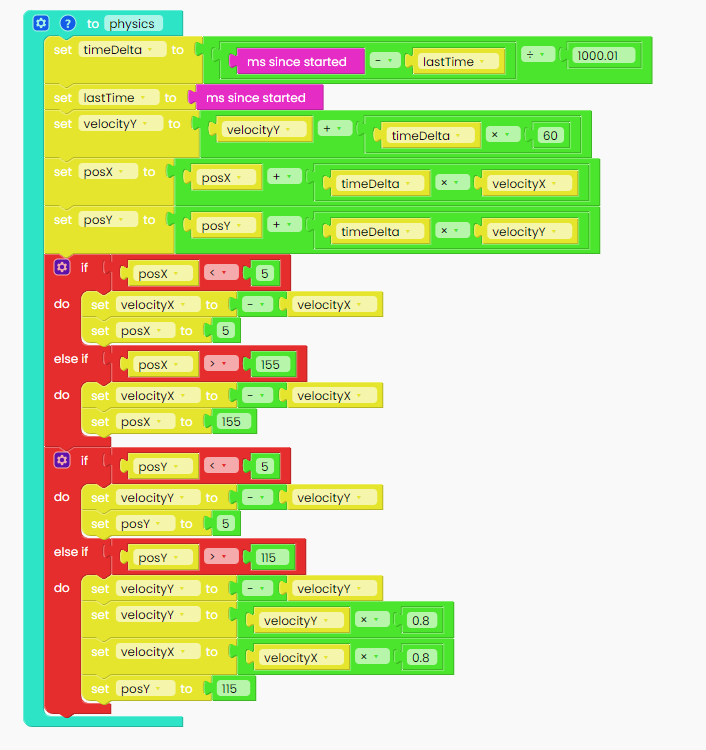As you can see, we did the same thing as we did with position X.

We've done the hard part! Congratulations!

Now, let's do some drawing.

Can you guess what the next step is?

That's right! Creating a new function - let's call it draw!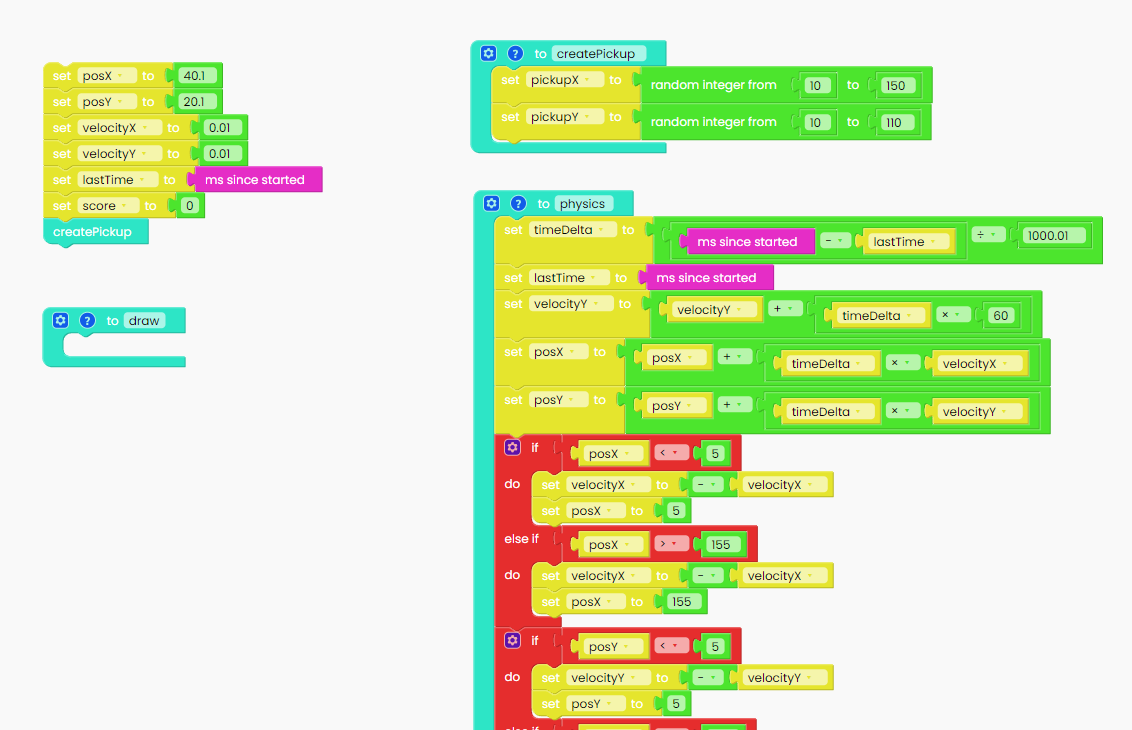Every time you draw something on your display, the first thing you want to do is fill the display with some color. We chose black.

You can find that particular block in the Display section.Once we color our screen black, we can start to draw.

As you already know, the game consists of a player represented by a ball and the other smaller balls that a player needs to catch.

Once again, let's go back to the Display section and choose one of the blocks.

This is the one you'll need:The x coordinate will be the variable "posX", and y coordinate will be the variable "posY".

is the size of the player ball, and we decided the size to be 4.

Now, let's draw the smaller balls you'll need to catch. The procedure is the same as we used to make a player ball.

Since we have to recognize two balls, we need to change the color of the other ball. You can put any color you want in your game.

Also, the x coordinate of the drawing function is variable "pickupX" and the y coordinate is the variable"pickupY".

As this is a smaller ball, its radius will be 3.Only one thing is left for us to draw, and that's the score. Making a game without keeping the score wouldn't make any sense.

You'll do that by dragging and dropping this particular block from the Display section:In order to display the score on the screen, we need to find the "create text" blue block.

We'll put the word "Score:" in the left circle, and for that, we need the first block from the Text section.

On the right side of the block, we'll put a variable called "score".

In x and y, you'll write the desired position where the score will be written on the screen.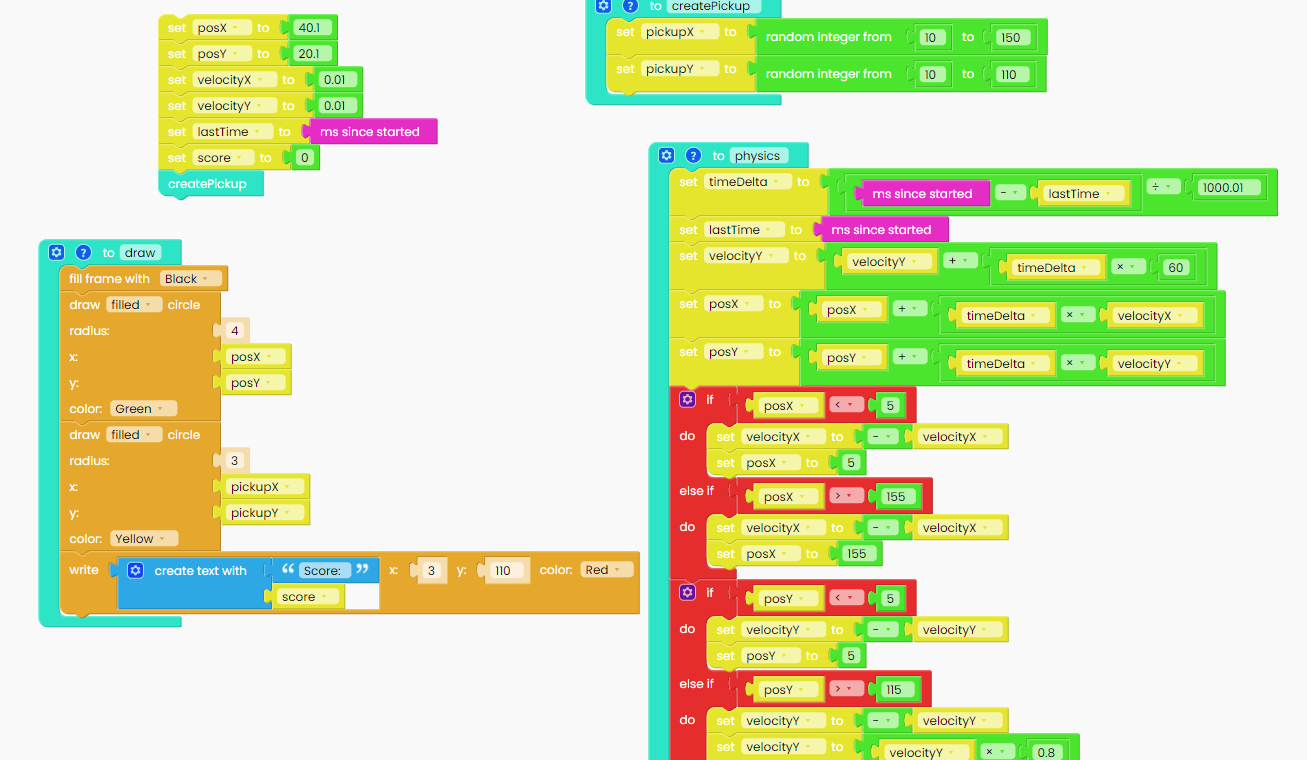Good job, we're done with drawing!

Create a new function, and call it "checkPickup". This function will detect whether the player has collected a ball.The first thing we'll have to do with this function is to calculate the distance between the player and the pickup ball. We used the formula you see below.

That's the formula you can use for calculating the distance between two points in a 2D space.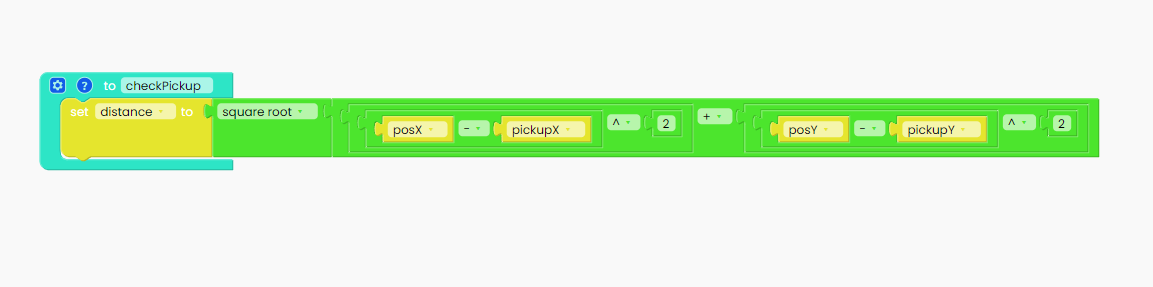We need to use the "if" block from the Logic section to check if the distance we mentioned is less than 8 pixels.

We'll use a comparison block to check that.If the distance is less than 8 pixels, that means that the player caught the ball, and your scoreboard increases by one point.

To keep the score, you'll have to take this block: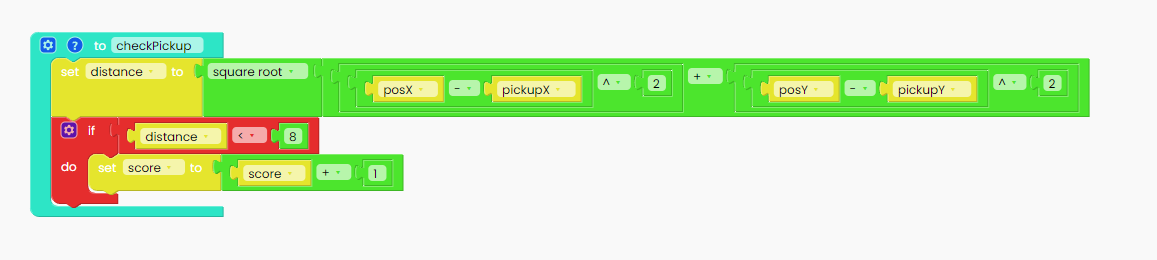So anytime you pick a ball, you'll get one point!

If you want to put your function in action, you need to call it. So, duplicate the "createPickup" block and drag it below.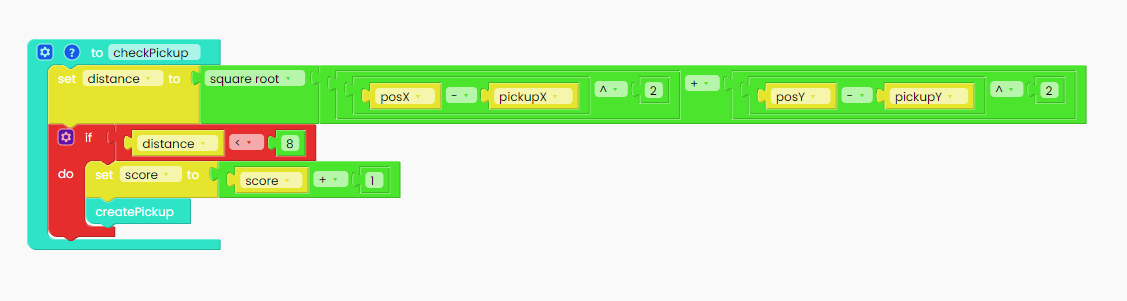The very last thing we'll do for our game is something we have already learned.

We'll use ByteBoi's pushbuttons to increase the velocity of the ball.

Open the I/O section, and click on the "When button up gets pressed" block.

If the right button gets pressed, velocityX will set to 70 in the positive direction,
and if the left button gets pressed, velocityX will be set to 70 in the negative direction on the x-axis.

If you press the button up, velocityY will change by 60.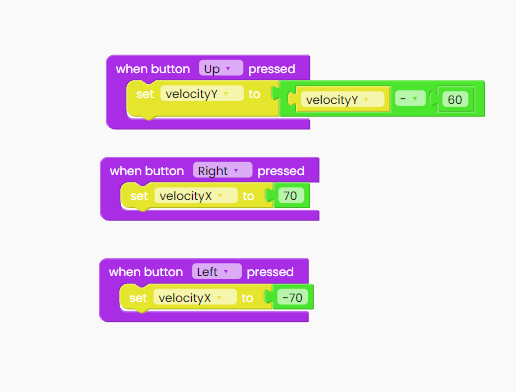At the end, don't forget to add the "Loop forever" block and inside it place the "physics", "checkPickup" and "draw" variables.

Also, add the "scan buttons" block.Complete game

This is it!

We tried to explain every part of the game, and now here it is all in one place.

We hope this tutorial has managed to help you make your first steps in video games creation.

Don’t forget to save and name your sketch.
Then, click the “Run” button and grab the ByteBoi!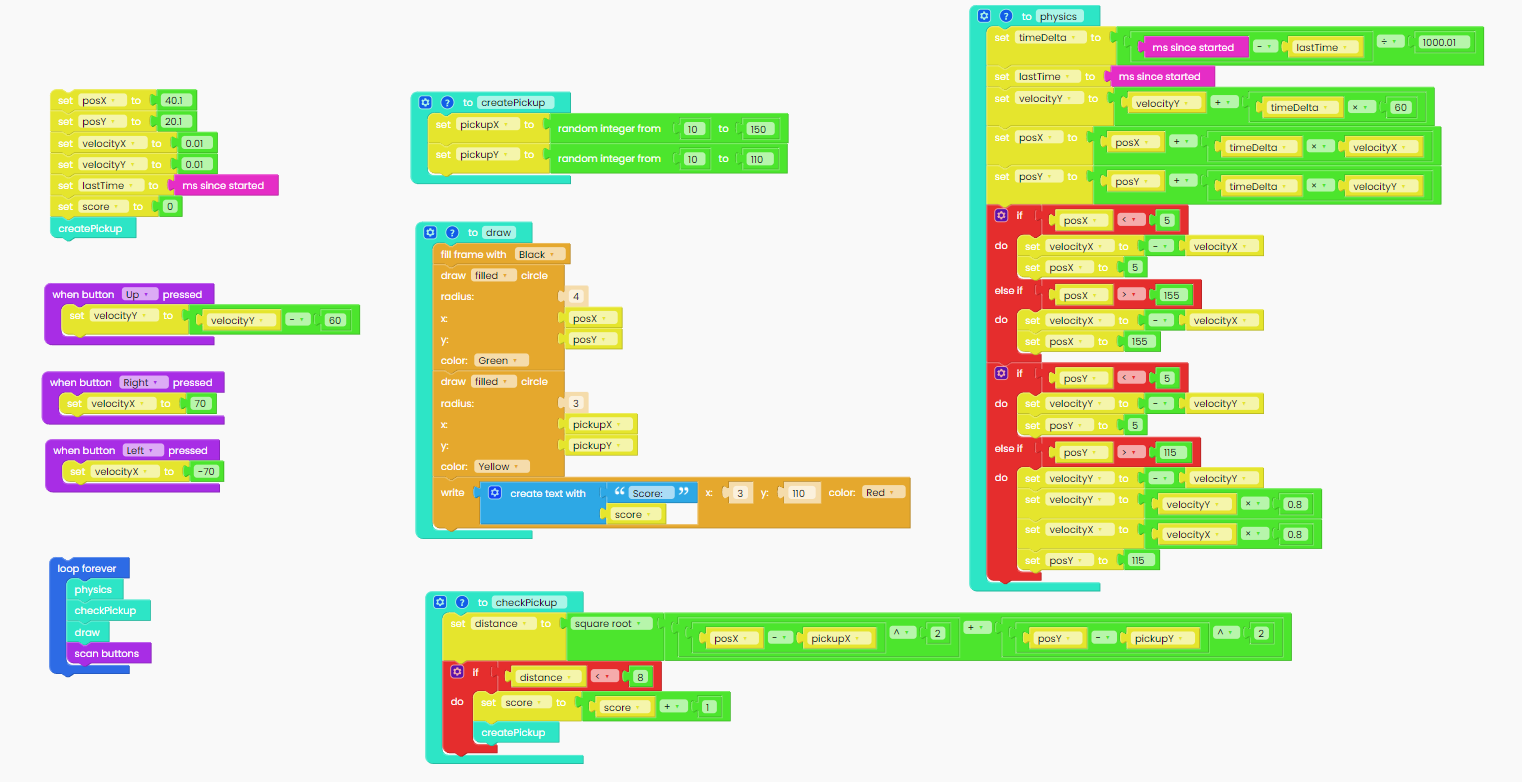Great job!